Vol 17, No 7 (2017) / Liu

Optical fiber positioning based on four-quadrant detector with Gaussian fitting method

Optical fiber positioning based on four-quadrant detector with Gaussian fitting method

Liu Jin-Sheng , Zou Hua *, Zhang Mei-Ling , Wang Lin-Zheng

College of Science, Hohai University, Nanjing 210098, China

† Corresponding author. E-mail: zouhua96@163.com

Abstract: Abstract

With the development of large-scale spectral surveys, fiber positioning technology has been developing rapidly. Because of the performance advantages of a four-quadrant (4Q) detector, a fiber positioning and real-time monitoring system based on the 4Q detector is proposed. The detection accuracy of this system is directly determined by the precision of the center of the spot. A Gaussian fitting algorithm based on the 4Q detector is studied and applied in the fiber positioning process to improve the calculated accuracy of the spot center. The relationship between the center position of the incident spot and the detector output signal is deduced. An experimental platform is built to complete the simulated experiment. Then we use the Gaussian fitting method to process experimental data, compare the fitting value with the theoretical one and calculate the corresponding error.

Keywords: instrumentation: detectors;methods: data analysis;techniques: imaging spectroscopy;telescopes

1 Introduction

Optical fiber technology has been widely used in the field of astronomical telescopes since optical fibers were first attached to telescopes in the 1980s. Due to their long distance transmission, ability to collect light in a useful range of diameters and flexible spatial arrangement, optical fiber technology can separate the terminal instrument from the focal plane of the telescope. These attributes greatly improve spectral acquisition rate and make large-scale astronomical spectroscopic surveys possible.

Large-scale astronomical spectroscopic surveys (Zhao ) (e.g., 2dF, 6dF, RAVE, SDSS, LAMOST and Gaia) have represented breakthroughs in astronomical observation technology by acquiring more abundant information on celestial bodies, which imaging surveys cannot provide. These efforts have obtained spectra of tens of millions of celestial bodies. The 2dF (2-degree Field Galaxy Redshift Survey) conducted by the Anglo-Australian Observatory, SDSS (Sloan Digital Sky Survey) based in the United States and LAMOST (Large Sky-Area Multi-Object Fiber Spectroscopic Telescope) headquartered in China (Cui et al. ) are three famous spectral survey projects.

An optical fiber positioning system is one of the key technologies in survey telescopes, which directly affect the observation efficiency and quality. 2dF adopts magnetic button positioning (McGrath et al. ), in which the light path turns 90° into the incident optical fiber through a small prism. A small magnet is placed beneath the prism and is attracted to the iron-based focal plane substrate by a robot and the fiber is lying on the focal plane redirecting the incident light into a spectrometer. As for the SDSS digital survey project, its optical fiber positioning relies on drilling holes in an about 500 mm aluminum plate according to pre-set coordinates (Smith et al. ), which are based on converting celestial coordinates to the focal plane plate. LAMOST adopts a parallel controllable fiber positioning technique. Four-thousand fiber positioning units are accommodated on the focal plane with a distance of 25.6 mm between them. Each unit drives a fiber moving in a circle 33 mm in diameter. The unit is driven by two stepper motors going through a double rotation movement. The maximum positioning error is 40 microns (Xing et al. ). In the motion process, the receiving end of an optical fiber does not generate deflection with respect to the optical axis of the telescope by always moving in the focal plane of the telescope without defocusing. The current optical fiber positioning system does not have a real-time monitoring and feedback system.

The four-quadrant (4Q) detector is a position sensitive instrument with wide dynamic and spectral ranges, high sensitivity, a fast response and small volume, as well as other advantageous characteristics. It is mainly used to capture, align and track a spot. With optimized performance of the 4Q detector, fiber positioning that incorporates a real-time monitoring system based on the 4Q detector is proposed. As celestial bodies that are targets of astronomical observation can be extremely dim, the detection accuracy of a fiber positioning system is directly determined by the accuracy of the spot center.

In this paper, a Gaussian fitting algorithm based on the 4Q detector is proposed and applied to fiber positioning to improve the detection accuracy of the center of the spot. First, it is necessary to model the relationship between the center position of the incident point and the output signal of the detector. Second, the associated experimental platform will be setup by implementing a Gaussian fitting method to deal with experimental data. Finally, we compare the fitted value with the theoretical one, and calculate the corresponding error. The results of this paper can guide practical application of this method.

2 Calculation of Spot Center

The 4Q detector is composed of four identical photoelectric detectors arranged in rectangular coordinates, separated by a cross-shaped channel in the middle (Lin et al. ). As shown in Figure 1, with the center of the detector as the coordinate origin, the spot is divided into four parts: A, B, C and D. In the following,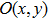represents the center of the incident spot; r the radius;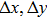offsets, I the corresponding quadrant of the output current; E, S the corresponding output current of the quadrant. The offset of the spot center can be determined through processing the input of the photocurrent signal and spot area (Feng & Deng ).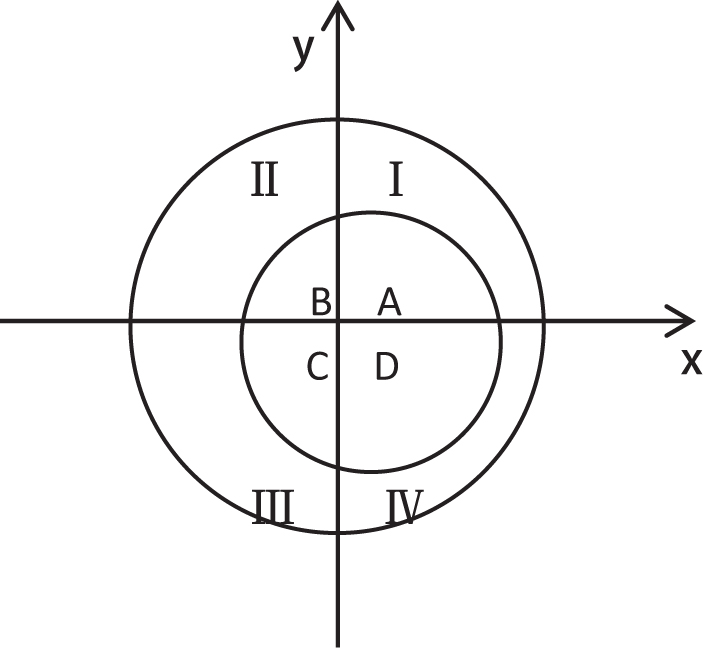Fig. 1 Diagram illustrating the principle of the 4Q detector.

The centroid algorithm is used to calculate the center position of the spot by the 4Q detector, and the spot is generally modeled as a uniform distribution, Gaussian distribution or Airy distribution (Zhou et al. ). In fact, stars are approximated as point sources, which are elliptical Gaussian spots in most circumstances (Zhao et al. ).

In this paper, according to the working principle of the 4Q detector and relevant properties of the spot, the relationship between the output current and the spot area is analyzed in detail, and the center of the spot can be calculated.

As the distance of the spot center from the origin is very small, x and y are far less than the radius of the spot. In the above formulas, respectively, apply an approximation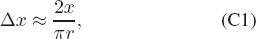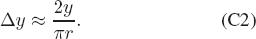That is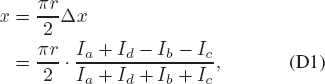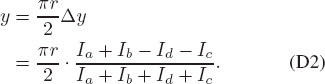After the 4Q detector data are fed back to the computer through the photoelectric position synthesis tester, the output current of the four electrodes can be read, and the center position of the spot can be directly obtained.

3 Gaussian Fitting Method for Calculating the Spot Center with the 4Q Detector

Methods for locating the spot center include the whole pixel positioning algorithm and the sub-pixel positioning algorithm. The sub-pixel positioning algorithm can be divided into an algorithm based on the edge and an algorithm based on the gray (Shen ). Bigger targets and those with gray boundaries obviously tend to use the ellipse fitting method and the Hough transform method based on the edge algorithm while smaller targets and gray fuzzy boundaries use the gray-based center localization algorithm, such as the barycenter method and the Gaussian fitting method.

The energy distribution of the laser spot is approximately consistent with a two-dimensional Gaussian distribution (Li et al. ; Fu et al. ), that is the intensity distribution follows a Gaussian function, and the gray-level boundary is fuzzy in the cross-section perpendicular to the beam. Therefore, the center of the spot is determined by the Gaussian fitting method based on a gray scale.

The 4Q detector and related software are used to record and read the coordinates. If the coordinates of points on the 4Q detector are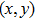, the corresponding value of gray is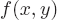.

We assume that the gray-scale distribution of a spot on the 4Q detector coincides with a two-dimensional Gaussian function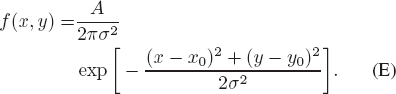In this expression,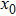,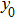are the center of the spot position;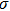is the standard deviation of the two-dimensional Gaussian function, which is related to defocus distance, object distance, focal length and so on. A is an undetermined coefficient, related to the properties of the spot. As can be seen from the above formula, the peak position is the center of the spot. Taking the logarithm of both sides of the above formula yields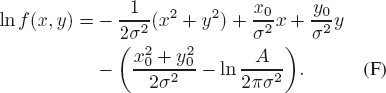Assume: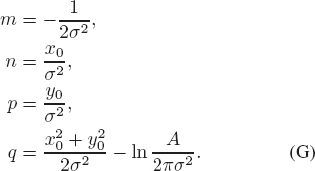After simplification, the original formula can be written as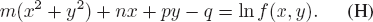Using the least squares principle, we solve the equation through a matrix transformation: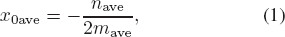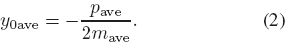where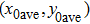is the center position of the spot obtained by using the Gaussian fitting method based on the 4Q detector.

4 Experiment and Analysis

4.1 Experimental Data

In a laboratory, an He-Ne laser is used to generate a star spot in the focal plane of the telescope. The spot is incident to the 4Q detector surface and the signal is transmitted to the optical fiber. Meanwhile, the signal is received by the signal receiving device. At the same time, the signal receiving device processes the signal generated by the detector, as shown in Figure 2. The experimental data are further processed and the effective gray information is extracted for Gaussian fitting to determine the center of the laser spot and provide necessary information for optical fiber positioning.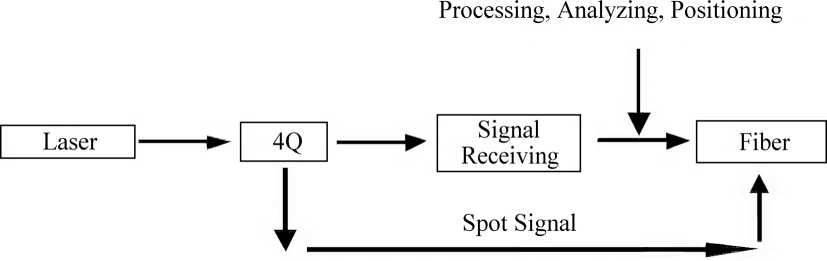Fig. 2 The process of optical fiber positioning based on the 4Q detector.

The unit used for 4Q detector position coordinates is millimeters. The raw data recorded by the 4Q detector are shown in Table 1.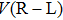is right to left intensity value of the 4Q detector,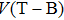the corresponding top to bottom value and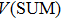their total relative intensity. X and Y are the instantaneous coordinates of the spot center considering the laser spot size and 4Q zero offset. In order to facilitate data processing and further reduce error, position coordinates of the detector are subdivided, using microns as the basic unit of data processing.

No.X Y
1 0.323 −0.326 0.287 6.491 −6.544
2 0.323 −0.329 0.288 6.488 −6.572
3 0.321 −0.346 0.281 6.568 −6.919
4 0.314 −0.326 0.296 6.249 −6.418
5 0.315 −0.316 0.308 6.087 −6.103
6 0.328 −0.347 0.285 6.611 −6.879
7 0.322 −0.338 0.281 6.575 −6.083
8 0.333 −0.322 0.287 6.634 −6.487
400 0.325 −0.325 0.291 6.464 −6.474

Notes: This entire table is available in the online version of the journal (http://www.raa-journal.org/docs/Supp/ms20160260Table1.doc).

Table 1 Raw Data Recorded by 4Q Detector

4.2 Data Dnalysis

In order to further validate accuracy of the Gaussian fitting method based on the 4Q detector, we caculate the error under the following condition. The sampling frequency parameters are as follows: 2.5 kHz, sampling range: ±1V, laser size: 4 mm; 4Q zero offset: (2 mm, −2 mm). Using MATLAB and Excel, we calculate the theoretical value and the Gaussian fitting value (unit: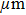) of the center of the spot (see Table 2). The relative intensity associated with the change in spot coordinate is shown in Figure 3.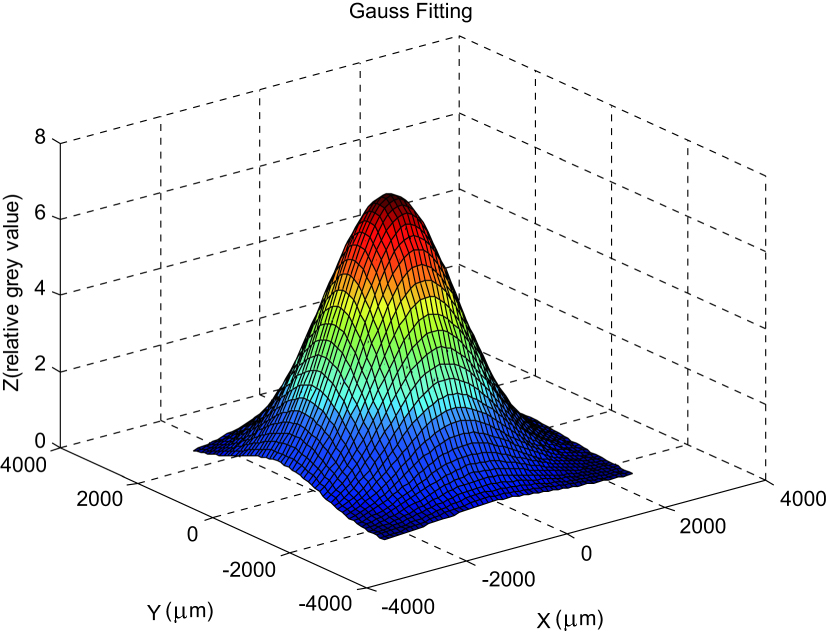Fig. 3 The change of the relative intensity of light spot with the coordinate.
Theoretical value Gaussian fitting Absolute errors
x 0 y 0 x 0 y 0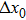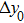255.6 −279.1 244.4 −269.0 11.2 10.1

Table 2 Results of the Two Methods and Their Absolute Errors

For the LAMOST telescope, 4000 fibers are placed at a radius of curvature of 20 m, with a 1.75 m diameter spherical focal panel, which is a separated positioning system, equidistantly spaced at 25.6 mm on the focal plane. Each unit positions a fiber over a range of of 33 mm. The incident end of the fiber must be able to achieve rapid and accurate positioning without blind spots on the surface of the focal plane. Therefore, the calculation accuracy of the algorithm should be better than 40to satisfy the engineering requirements. Considering the actual tolerance distribution, they should be better than 20to fully meet the real needs.

By analyzing the data in Table 2, it can be seen that the center position with the Gaussian fitting method of the spot exactly matches the theoretical value, which satisfies requirements for the LAMOST telescope in terms of positioning error.

5 Conclusions

In this paper, a Gaussian fitting method based on the 4Q detector is proposed. In addition, by applying the theory describing the relationship between coordinates of the spot center and 4Q detector input signals, an experimental platform was simulated, and position of the spot center was fitted. Compared with the existing fiber positioning method, it has the following advantages: acquiring data quickly and accurately, real-time monitoring feedback, high precision, good stability and easy operation. In the future, this technology can be efficiently implemented in the LAMOST telescope spherical focal panel fiber positioning system.

 Cui X.-Q. Zhao Y.-H. Chu Y.-Q. et al. 2012 RAA(Research in Astronomy and Astrophysics) 12 1197 Feng L.L. Deng R.L 1996 Infrared and Laser Engineering 25 16 (in Chinese) Fu R.-G. Chang B.-K. Liu S.-R. 2004 Optoelectronic Technology & Information 17 26 Li L. Gong M.-L. Liu X.-Z. et al. 2000 Laser Technology 24 405 Lin Z.-Q. Li H.-J. Lang Y. H. Yin F.-C. 2009 Optics and Precision Engineering 17 764 McGrath A. Barden S. Miziarski S. Rambold W. Smith G. 2008 SPIE Astronomical Telescopes+Instrumentation International Society for Optics and Photonics 70144K Shen J. 2009 Research on Sub-pixel Edge Location Algorithm Based on Moment PhD thesis Chongqing Univ. of Posts and Telecommunications Smith G. Brzeski J. Miziarski S. et al. 2004 SPIE Astronomical Telescopes+Instrumentation International Society for Optics and Photonics 5495 173 Xing X.-Z. Hu H.-Z. Du H.-S. et al. 1997 Journal of China University of Science and Technology 27 492 Zhao X. Tong S.-f. Jiang H.-l. 2010 Optics and Precision Engineering 10 2164 Zhao Y.-H. 2014 Scientia Sinica Physica, Mechanica & Astronomica 44 1041 Zhou G. Chu X. Zheng J. 2008 Chinese Optics Letters 6 395
Cite this article: Liu Jin-Sheng, Zou Hua, Zhang Mei-Ling, Wang Lin-Zheng. Optical fiber positioning based on four-quadrant detector with Gaussian fitting method. Res. Astron. Astrophys. 2017; 7:075.

Refbacks

• There are currently no refbacks.